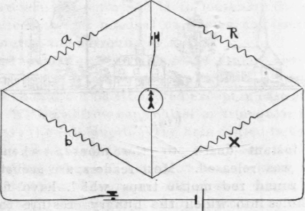Oscar N. Dame

Measurements of resistance are made by comparing the unknown resistance to be measured with certain standards of known resistance, there being several different methods in general use. The standard resistance coils are made of German silver or platinum alloy, as these metals have high resistance, which varies less than other metals, with change of temperature. Resistance boxes are constructed with a large number of coils of varying resistances, so that by means of plugs about any desired resistance can be obtained.The Wheatstone Bridge

One of the most commonly used methods is that of X = unknown Resistance.

a and b are fixed resistances in proportion to one another; for instance, as 1 to 100 or 1 to 1,000.

R= an adjustable resistance which should be adjusted until there is no galvanometer deflection with both keys depressed.

Then this ratio exists:

A : b :: R : x or ax = bR, that is, the products of opposite sides of the bridge are always equal to each other.

Should a and b be equal, then x=R.

Should a : b = 1 : 100, then x = 100 times R.

Often further accuracy is required. For example: Let a=10andb =1,000; x = between 5.11 and 5.12

Notice the galvanometer deflection to left and right and less resistances in "R " and calculate by proportion the excess of the true resistance over the lesser.

R=5.11=for instance, 6 deflection to left = d1.

R=5.12 = for instance, 12 deflection to right = d2.

Then(d1+d2) :l::d1:y that is (6+12) : 1 ::6:y that is (18 :1 ::6 :y = about .3.

hence x = 5.113.